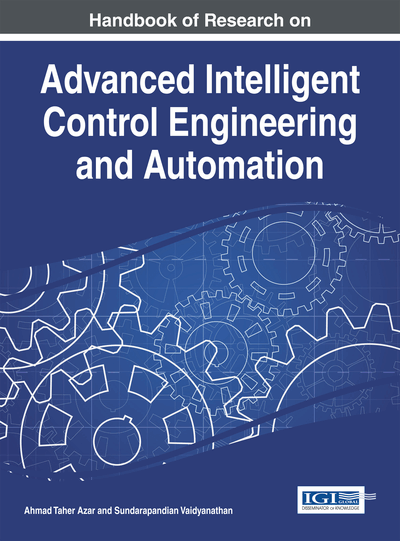# Chaos Control of an Impact Mechanical Oscillator Based on the OGY Method

Hassène Gritli (Institut Supérieur des Etudes Technologiques de Kélibia, Tunisia), Safya Belghith (Ecole Nationale d'Ingénieurs de Tunis, Tunisia) and Nahla Khraief (Ecole Supérieure de Technologie et d'Informatique, Tunisia)
DOI: 10.4018/978-1-4666-7248-2.ch009

## Abstract

This chapter deals with the control of chaos exhibited in the behavior of a one-degree-of-freedom impact mechanical oscillator with a single rigid constraint. The mathematical model of such impact oscillator is represented by an impulsive hybrid linear non-autonomous dynamics. The proposed control approach is based chiefly on the OGY method. First, an analytical expression of a constrained controlled Poincaré map is derived. Secondly, the linearized controlled Poincaré map around the fixed point of the constrained map is determined. Relying on the linearized map, a state feedback controller is designed. It is shown that the proposed control strategy is efficient for the control of chaos for the desired fixed point and for the fixed parameter. It is shown also that, by changing the system parameter, the behavior of the impact mechanical oscillator changes radically. Thus, the drawback of the developed OGY control method is revealed and some remedies are given.
Chapter Preview
Top

## 1. Introduction

Chaos is defined as a strange phenomenon with erratic appearance and which arises in deterministic continuous or discrete nonlinear systems. The chaos paradigm provides a better understanding of the inherent properties of natural systems. A chaotic system is a nonlinear dynamic system and its response has certain characteristics:

• It has an excessive sensitivity to initial conditions: the very small difference between the same two initial conditions will grow and the two paths from these two initial conditions separate exponentially over time.

• A chaotic system has a wide spectrum of the Fourier transform, and has fractal properties of the motion in the phase space.

• In addition, the chaotic motion of a dynamical system is locally unstable and globally bounded in the phase space. This means that solutions with very similar initial conditions will diverge at a certain distance after a finite time. Thus, chaos denotes a state of disorder and irregularity which reflects that the forecast is only possible in the short term.

• Moreover, a nonlinear chaotic dynamic system is said to be chaotic if it has at least one strange attractor. An attractor is called strange or chaotic if it is bounded and all trajectories starting from this attractor are actually chaotic (Andrievskii & Fradkov, 2003; Fradkov & Evans, 2005).

The study of nonlinear science has evolved into a new era. Fundamental theories have been established and important results have been obtained in the areas of chaotic nonlinear dynamics such as chaos control, namely, the investigation of bringing order to chaos (Wiggins, 2003; Schöll & Schuster, 2007). It is now recognized that chaos occurs commonly in many fields of science and engineering and so in a large variety of nonlinear dynamical systems such as nonlinear oscillators (Lakshmanan & Murali, 1996; Fradkov & Pogromsky, 1996), impact mechanical systems (Wiercigroch & de Kraker, 2000; Awrejcewicz & Lamarque, 2003; Thota & Dankowicz, 2006a, 2006b; Thotaa et al., 2006; Jin et al., 2006), legged walking robots (Kaygisiz et al., 2006; Gritli et al., 2012a, 2012b, 2012c, 2014b; Iqbal et al., 2014), etc. Nonlinear dynamics of impact oscillators and their nonlinear behaviors have received considerable theoretical and experimental attentions in the past. Many mathematical-numerical studies of these impacting systems have been carried out, and particular interest has been focused on the analysis and the control of chaos and bifurcations (Lenci & Rega, 2004). More general studies of multiple-degrees-of-freedom impact mechanical oscillators have also been carried out (Pfeiffer & Glocker, 1996; Pun et al., 1998; Wagg, 2005). However, almost all these studies focus primarily on a single impact constraint. In this chapter, we will consider a one-degree-of-freedom impact mechanical oscillator periodically excited by an external sinusoidal input. This oscillator consists of one mass, coupled with one spring and one dashpot, and the motion of the mass is restricted by only one rigid constraint (Budd, 1996; de Souza & Caldas, 2004a, 2004b; de Souza et al., 2007). This proposed impact system is classified as an impulsive hybrid linear non-autonomous dynamics that is simple enough to be amendable to analysis, yet complex enough to exhibit a wealth of attractive phenomena.

## Key Terms in this Chapter

LMI: Linear Matrix Inequality.

Hybrid Poincaré Map: A discrete application that is constrained with algebraic conditions.

Linearized Poincaré Map: A Poincaré map linearized around a fixed point.

Chaos: A nonlinear erratic phenomenon that is found to be exhibited in several physical systems.

Hybrid Limit Cycle: A periodic behavior of a dynamical system that has a transition in the state vector.

Impact Mechanical Oscillator: A mechanical physical system that undergoes impacts with a rigid wall.

OGY Control: A control method that was invented by Ott, Grebogi andYorke in 1990 to control chaos in nonlinear dynamics.

## Complete Chapter List

Search this Book:
Reset